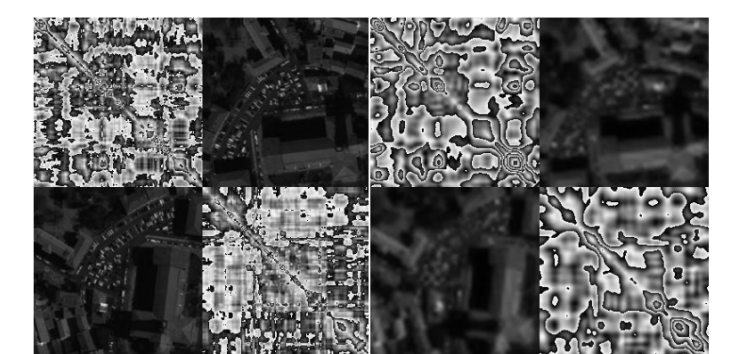Currently the Riemannian geometry of symmetric positive definite (SPD) matrices is gaining momentum as a powerful tool in a wide range of engineering applications such as image, radar and biomedical data signal processing. If the data is not natively represented in the form of SPD matrices, typically we may summarize them in such form by estimating covariance matrices of the data. However once we manipulate such covariance matrices on the Riemannian manifold we lose the representation in the original data space. For instance, we can evaluate the geometric mean of a set of covariance matrices, but not the geometric mean of the data generating the covariance matrices, the space of interest in which the geometric mean can be interpreted. As a consequence, Riemannian information geometry is often perceived by non-experts as a “black-box” tool and this perception prevents a wider adoption in the scientific community. Hereby we show that we can overcome this limitation by constructing a special form of SPD matrix embedding both the covariance structure of the data and the data itself. Incidentally, whenever the original data can be represented in the form of a generic data matrix (not even square), this special SPD matrix enables an exhaustive and unique description of the data up to second-order statistics. This is achieved embedding the covariance structure of both the rows and columns of the data matrix, allowing naturally a wide range of possible applications and bringing us over and above just an interpretability issue. We demonstrate the method by manipulating satellite images (pansharpening) and event-related potentials (ERPs) of an electroencephalography brain-computer interface (BCI) study. The first example illustrates the effect of moving along geodesics in the original data space and the second provides a novel estimation of ERP average (geometric mean), showing that, in contrast to the usual arithmetic mean, this estimation is robust to outliers. In conclusion, we are able to show that the Riemannian concepts of distance, geometric mean, moving along a geodesic, etc. can be readily transposed into a generic data space, whatever this data space represents.